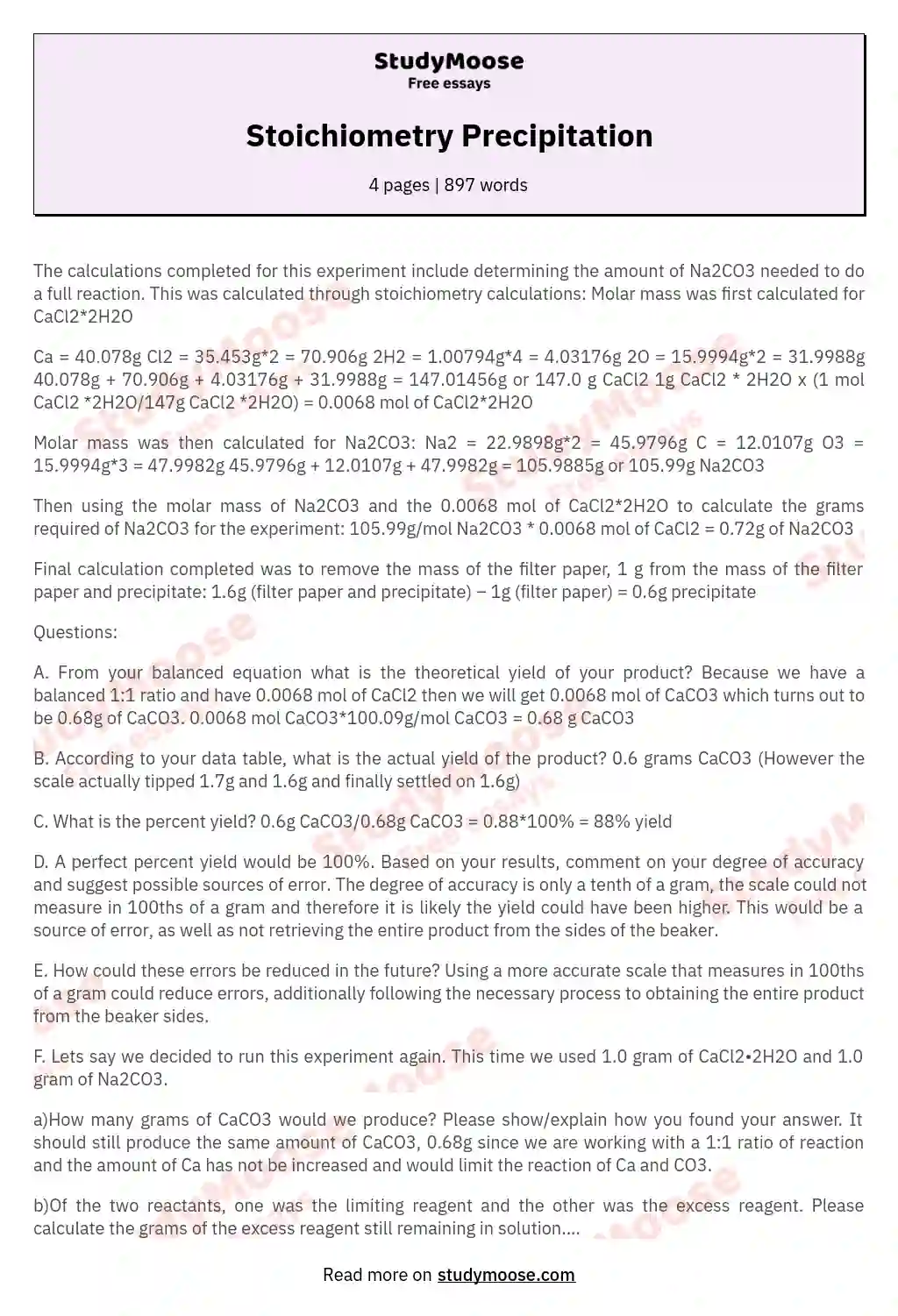# Stoichiometry Precipitation

The calculations completed for this experiment include determining the amount of Na2CO3 needed to do a full reaction. This was calculated through stoichiometry calculations: Molar mass was first calculated for CaCl2*2H2O

Ca = 40.078g Cl2 = 35.453g*2 = 70.906g 2H2 = 1.00794g*4 = 4.03176g 2O = 15.9994g*2 = 31.9988g 40.078g + 70.906g + 4.03176g + 31.9988g = 147.01456g or 147.0 g CaCl2 1g CaCl2 * 2H2O x (1 mol CaCl2 *2H2O/147g CaCl2 *2H2O) = 0.0068 mol of CaCl2*2H2O

Molar mass was then calculated for Na2CO3:
Na2 = 22.9898g*2 = 45.

9796g C = 12.0107g O3 = 15.9994g*3 = 47.9982g 45.9796g + 12.0107g + 47.9982g = 105.9885g or 105.99g Na2CO3

Then using the molar mass of Na2CO3 and the 0.0068 mol of CaCl2*2H2O to calculate the grams required of Na2CO3 for the experiment: 105.99g/mol Na2CO3 * 0.0068 mol of CaCl2 = 0.72g of Na2CO3

Final calculation completed was to remove the mass of the filter paper, 1 g from the mass of the filter paper and precipitate: 1.6g (filter paper and precipitate) – 1g (filter paper) = 0.

Get quality help nowDoctor JenniferVerified writer

Proficient in: Aspirin5 (893)

“ Thank you so much for accepting my assignment the night before it was due. I look forward to working with you moving forward ”+84 relevant experts are online

6g precipitate

Questions:

A. From your balanced equation what is the theoretical yield of your product? Because we have a balanced 1:1 ratio and have 0.0068 mol of CaCl2 then we will get 0.0068 mol of CaCO3 which turns out to be 0.68g of CaCO3. 0.0068 mol CaCO3*100.09g/mol CaCO3 = 0.68 g CaCO3

B. According to your data table, what is the actual yield of the product? 0.6 grams CaCO3 (However the scale actually tipped 1.7g and 1.6g and finally settled on 1.6g)

C. What is the percent yield?
0.6g CaCO3/0.68g CaCO3 = 0.88*100% = 88% yield

D. A perfect percent yield would be 100%. Based on your results, comment on your degree of accuracy and suggest possible sources of error. The degree of accuracy is only a tenth of a gram, the scale could not measure in 100ths of a gram and therefore it is likely the yield could have been higher. This would be a source of error, as well as not retrieving the entire product from the sides of the beaker.

E. How could these errors be reduced in the future?
Using a more accurate scale that measures in 100ths of a gram could reduce errors, additionally following the necessary process to obtaining the entire product from the beaker sides.

F. Lets say we decided to run this experiment again. This time we used 1.0 gram of CaCl2•2H2O and 1.0 gram of Na2CO3.

a)How many grams of CaCO3 would we produce? Please show/explain how you found your answer. It should still produce the same amount of CaCO3, 0.68g since we are working with a 1:1 ratio of reaction and the amount of Ca has not be increased and would limit the reaction of Ca and CO3.

b)Of the two reactants, one was the limiting reagent and the other was the excess reagent. Please calculate the grams of the excess reagent still remaining in solution. The limiting reagent would be the CaCl2. Since we only need 0.72g of Na2CO3(see calculations and erros section above) and are now working with 1g we should have an excess of 0.28g of Na2CO3. 1g Na2CO3 – 0.72g Na2CO3 =0.28g Na2CO3

G. Before the advent of Advil and Tylenol, did people simply have to “grin and bear it” when it came to pain? One of the most common ancient medicines for pain, fever, and inflammation came as a byproduct of the willow tree. While the first uses date back to 400 BCE, American historians cite the use of willow bark tea by the Lewis and Clark exploration party in the early 1800’s. Salicylic acid derived from the willow tree’s bark was the key chemical involved with the relief of pain and the reaction to make aspirin is a fairly simple one performed in numerous chemistry classroom nation wide. Aspirin can be made by reacting acetic anhydride (C4H6O3) with salicylic acid (C7H6O3) to form aspirin (C9H8O4).

C4H6O3 + C7H6O3 –> C2H4O2¬ + C9H8O4

When synthesizing aspirin, a student began with 3.20 mL of acetic anhydride (density = 1.08 g/mL) and 1.45 g of salicylic acid. The reaction was allowed to run its course and 1.23 grams of aspirin was collected by the student. Determine the limiting reactant, theoretical yield of aspirin and percent yield for the reaction.

Molar mass calculations:
C4H6O3:
C4 = 12.0107g*4 = 48.0428g H6 = 1.00794g*6 = 6.04764g O3 = 15.9994*3 = 47.9982g 48.0428g + 6.04764g + 47.9982g = 102.07244g or 102.07g of C4H6O3

C7H6O3:
C7 = 12.0107g*7 = 84.0749g H6 = 1.00794g*6 = 6.04764g O3 = 15.9994*3 = 47.9982g 84.0749g + 6.04764g + 47.9982g = 138.12074g or 138.12g C7H6O3

C9H8O4:
C9 = 12.0107g*9 = 108.3663g H8 = 1.00794g*8 = 8.06352g O4 = 15.9994*4 = 63.9976g 108.3663g + 8.06352g + 63.9976g = 180.42742g or 180.43g C9H8O4

Using the information provided we calculate the grams of acetic anhydride: 3.20mL*1.08 g/mL = 3.456 g of acetic anhydride (C4H6O3)

Then we need to calculate the moles of each reagent:
3.46g C4H6O3/102.07g/mol of C4H6O3 = 0.0339 mol C4H6O3
1.45g C7H6O3/138.12g C7H6O3 = 0.0105 mol C7H6O3

Because we have a 1:1 ratio then we can determine that our limiting reagent is the C7H6O3, salicylic acid

The theoretical yield should be calculated next:
0.0105 mol C7H6O3 x 180.43g/mol C9H8O4 = 1.89 g of C9H8O4 is our theoretical yield

Then the percent yield should be calculated:
1.23g C9H8O4/1.89g C9H8O4 = 0.65 x 100% = 65% yield